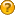###Author Topic: residual floating window  (Read 2739 times)

#### attila homoki

• Newbie
•• Posts: 4
• Karma: +0/-0##### residual floating window
« on: July 26, 2017, 04:26:27 am »
Hi PTL Team,

I'm trying to come up with a matlab script to visualize my PTL residual model positions.
So my aim is to create the same zscore graph as you did on the backtesting result page (residual model -> Z-Score graph).

I have no problem with the OLS and to create the residual chart (for the whole timerange) of both stocks - as seen on the Orthogonal Residuals and Analysis of Residuals graphs on the analysis page.

But when i'm following the steps of the wiki page model description, I don't see how to apply the  floating window and how to "calculate the regression for each day" ?

When I iterate through on the two stock time series, A(t) and B(t) and calculate a regression on each day (for the past or next period of 'P' days), then I will end up with T-P number of residual time series (all of them have P length) .

How do you process this result into one final residual time series ?

Can you please explain that part of the model description a bit more in detail?

thank you !

• Sr. Member
••• Posts: 315
• Karma: +12/-0##### Re: residual floating window
« Reply #1 on: July 28, 2017, 10:08:08 am »
Hi Attila,

the wiki describes the process correctly.
1) you have A(t) and B(t)
2) for each sample (day) you calculate a linear regression for last P days - so for each t you get two regression coefficients (alpha and beta) and - you are correct - you also get P values of residuals for each day...but you only take the LAST residual - so for each t you have one alpha(t), one beta(t) and one R(t)

So I guess the confusion is about residuals - you only take the LAST residual for each day

Kind regards,
Karel

#### attila homoki

• Newbie
•• Posts: 4
• Karma: +0/-0##### Re: residual floating window
« Reply #2 on: July 31, 2017, 09:25:10 pm »
Hi Karel, I could re-create the plot by keeping only the last residual of the p-window, thanks!